## Maximum Power Transfer Theorem:

Here, the maximum power transfer theorem has been discussed for resistive loads. The maximum power transfer theorem states that the maximum power is delivered from a source to its load when the load resistance is equal to the source resistance. It is for this reason that the ability to obtain impedance matching between circuits is so important.

For example, the audio output transformer must match the high impedance of the audio power amplifier output to the low input impedance of the speaker. Maximum Power Transfer is not always desirable, since the transfer occurs at a 50 per cent efficiency.

In many situations, a maximum voltage transfer is desired which means that unmatched impedances are necessary. If maximum power transfer is required, the load resistance should equal the given source resistance. The maximum power transfer theorem can be applied to complex impedance circuits. If the source impedance is complex, then the maximum power transfer occurs when the load impedance is the complex conjugate of the source impedance

Consider the circuit shown in Fig. 7.31, consisting of a source impedance delivering power to a complex load.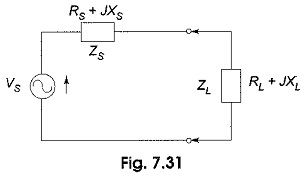Current passing through the circuit shown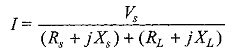Magnitude of current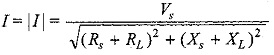Power delivered to the circuit is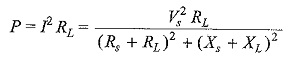In the above equation, if RL is fixed, the value of P is maximum when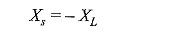Then the power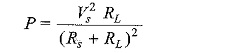Let us assume that RL is variable. In this case, the maximum power is transferred when the load resistance is equal to the source resistance. If RL = Rs and XL = -Xs, then ZL = Z*s. This means that the Maximum Power Transfer occurs when the load impedance is equal to the complex conjugate of source impedance Zs.

Scroll to Top